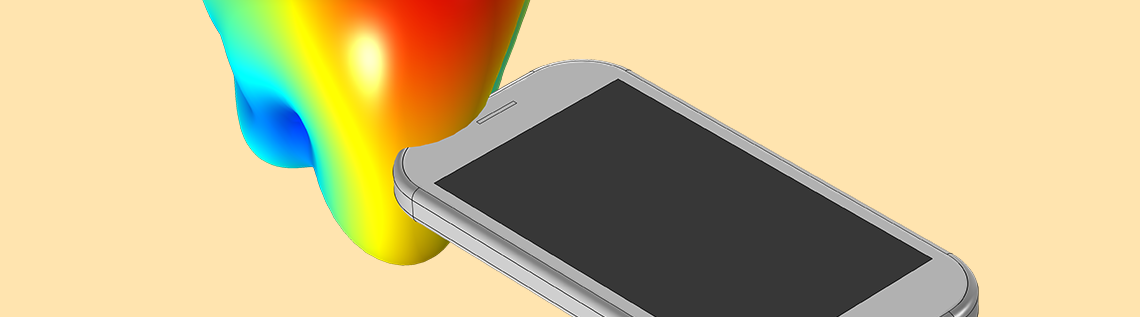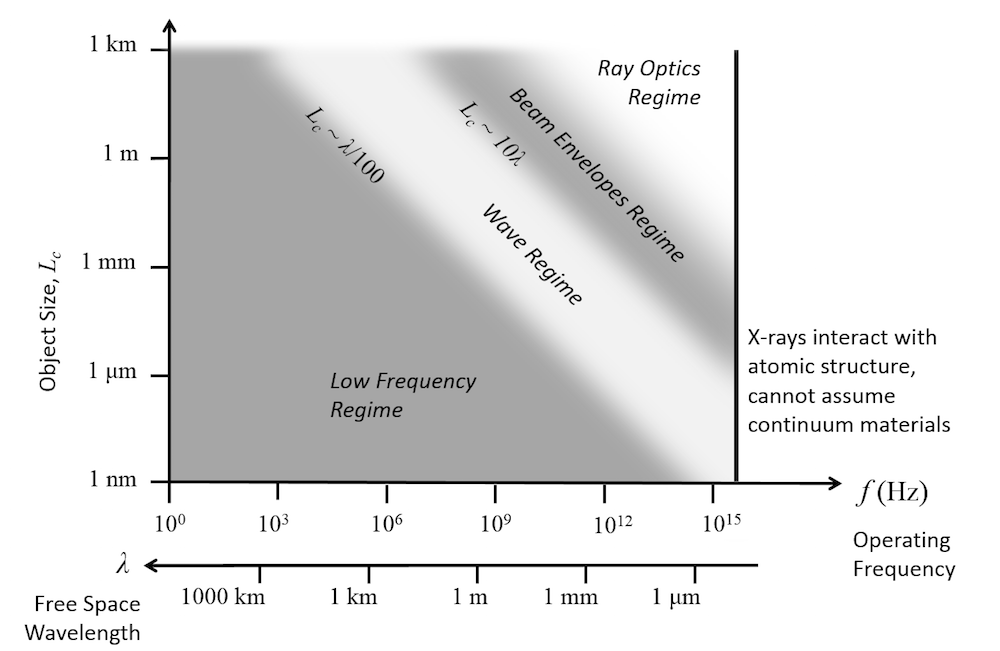# 在 COMSOL 中可以使用哪个模块进行电磁学模拟？

2020年 7月 28日

### 计算电磁学：麦克斯韦方程组

 \nabla \cdot \mathbf{D} = \rho \nabla \cdot \mathbf{B} = 0 \nabla \times \mathbf{E} = -\frac{\partial}{\partial t} \mathbf{B} \nabla \times \mathbf{H} = \mathbf{J} +\frac{\partial}{\partial t}\mathbf{D}

### 稳态电场模拟

\nabla \cdot \left( – \sigma \nabla V \right ) = 0

\nabla \cdot \left( – \epsilon \nabla V \right ) = 0

### 时域和频域电场模拟

\nabla \cdot \left( \mathbf{J_c +J_d} \right ) = 0

}{\partial t}
。当源信号不是谐波，并且我们希望随时间监视系统响应时，可以使用此方法。电路中电容器的瞬态模拟模型是一个你可以查阅的示例。

\nabla \cdot \left( – \left( \sigma + j \omega \epsilon \right) \nabla V \right ) = 0

### 使用 AC/DC 模块模拟磁场

AC/DC 模块解决了稳态、时域或低频状态下的磁场模拟问题。

\nabla \cdot \left( – \mu \nabla V_m \right ) = 0

\nabla \times \left( \mu ^ {-1} \nabla \times \mathbf{A} \right)= \mathbf{J}

\nabla \times \left( \mu ^ {1} \nabla \times \mathbf{A} \right)= \sigma \frac{ \partial \mathbf{A}}{\partial t}

\nabla \times \left( \mu ^ {-1} \nabla \times \mathbf{A} \right) = -\left( j \omega \sigma – \omega^2 \epsilon \right) \mathbf{A}

### 使用 RF 模块或波动光学模块模拟频域和时域中的波动方程

\nabla \times \left( \mu_r ^ {-1} \nabla \times \mathbf{E} \right) -\omega^2 \epsilon_0 \mu_0 \left(\epsilon_r – j \sigma/\omega \epsilon_0 \right) \mathbf{E} = 0

\nabla \times \left( \mu_r ^ {-1} \nabla \times \mathbf{A} \right)+ \mu_0 \sigma \frac{ \partial \mathbf{A}}{\partial t} +\mu_0 \frac{ \partial}{\partial t}\left( \epsilon_0 \epsilon_r \frac{ \partial \mathbf{A}}{\partial t} \right) = 0

\left( \nabla – i \nabla \phi \right) \times \mu_r ^ {-1} \left( \left( \nabla – i \nabla \phi \right) \times \mathbf{E_e} \right) -\omega^2 \epsilon_0 \mu_0 \left(\epsilon_r – j \sigma/\omega \epsilon_0 \right) \mathbf{E_e} = 0

### 在 AC/DC 模块、RF 模块和波动光学模块之间选择

AC/DC 模块和 RF 模块之间的分界线有点模糊。问我们自己几个问题会有所帮助：

1. 我正在使用的设备会辐射大量能量吗？我对计算谐振感兴趣吗？如果是这样，则RF模块更合适。
2. 设备是否比最高工作波长的波长小得多？我主要对磁场感兴趣吗？如果是这样，则 AC/DC 模块更合适。